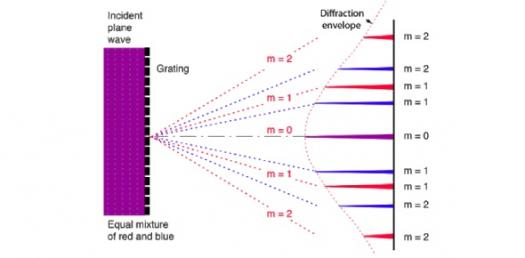# Diffraction Grating - Light Experiment Quiz

Approved & Edited by ProProfs Editorial Team
At ProProfs Quizzes, our dedicated in-house team of experts takes pride in their work. With a sharp eye for detail, they meticulously review each quiz. This ensures that every quiz, taken by over 100 million users, meets our standards of accuracy, clarity, and engagement.
| Written by Kramayp
K
Kramayp
Community Contributor
Quizzes Created: 4 | Total Attempts: 7,965
Questions: 10 | Attempts: 1,465SettingsA Diffraction grating is an optical unit with a periodic structure that diffracts and splits light. A short quiz on the key concepts in the Diffraction Gratings Unit. Just type in your name and start the Diffraction Grating-Light Experiment quiz. Answers to the questions will be presented after you attempt the quiz question. Best of luck!

• 1.

### Why are diffraction gratings used instead of single slit or double slits to measure the wavelength of light?

• A.

Gratings provide a much wider diffraction maxima.

• B.

Gratings provide equal spacing between the maxima and minima.

• C.

Gratings provide very narrow diffraction maxima which can be accurately measured.

• D.

Both A and B

• E.

None of the Above

C. Gratings provide very narrow diffraction maxima which can be accurately measured.
Explanation
Since there are thousands of slits per centimeter in a diffraction grating, there is a lot more destructive interference. This means that the diffraction peaks/maximas are a lot narrower and can therefore be accurately measured.

Rate this question:

• 2.

### For diffraction gratings, 'd' represents

• A.

Spaces between antinodes in a diffraction pattern

• B.

Width of each one of the slits in the grating.

• C.

The distance between the grating and the screen.

• D.

Distance between each one of the slits in the grating

• E.

Both A and B

B. Width of each one of the slits in the grating.
Explanation
Interfringe stands for the spaces between the nodes or the antinodes in a double slit diffraction pattern.

Rate this question:

• 3.

### When white light passes through a diffraction grating, there are places where some of the colours in the diffracted light overlap.

• A.

True

• B.

False

A. True
Explanation
Since different colours in white light have different wavelengths, the minima and maxima for different colours occur at different distances from the screen centre, and thus, can overlap. Also, colours with greater wavelengths diffract more than those with shorter wavelengths.

Rate this question:

• 4.

### At what angle will 560-nm light produce a second-order maximum when falling on a grating whose slits are 1.45 X 10^-3 cm apart?

• A.

12.2 degrees

• B.

4.4 degrees

• C.

32.4 degrees

• D.

14.8 degrees

• E.

None of the Above

B. 4.4 degrees
Explanation
Solution to a similar question is given on the diffraction grating sample problems section on the website.

Rate this question:

• 5.

### For diffraction gratings, 'd' is calculated by dividing the number of slits by the length of the grating (in meters)

• A.

True

• B.

False

B. False
Explanation
'd' is calculated by dividing the length of the grating (in meters) by the number of slits. This gives the distance between each slit.

Rate this question:

• 6.

### A full spectral order refers to an order in which all the colours/wavelengths of light are visible.

• A.

True

• B.

False

A. True
Explanation
A full spectral order refers to an order in which all the colors/wavelengths of light are visible. This means that in a full spectral order, all the colors of the visible spectrum are present and can be seen. Therefore, the statement "True" is correct as it accurately describes a full spectral order.

Rate this question:

• 7.

### Diffraction Gratings follow the same mathematical model as Single Slit Diffraction

• A.

True

• B.

False

B. False
Explanation
Diffraction Gratings follow the same mathematical model as Double Slit Diffraction.

Rate this question:

• 8.

### The farther apart the slits in a grating, the wider the diffraction pattern.

• A.

True

• B.

False

B. False
Explanation
The farther apart the slits, the narrower the diffraction pattern because the K max becomes smaller and therefore less diffraction occurs.

Rate this question:

• 9.

### What is the formula for diffraction grating?

• A.

D = \frac {1} { N }

• B.

D = \frac {1/N }

• C.

D = {2} { N }\frac

A. D = \frac {1} { N }
Explanation
The formula for diffraction grating is d = 1/N. This formula represents the relationship between the spacing of the grating (d) and the number of lines per unit length (N). It states that the spacing of the grating is inversely proportional to the number of lines per unit length. As the number of lines per unit length increases, the spacing between the lines decreases. This formula is commonly used in physics and optics to calculate the properties of diffraction gratings.

Rate this question:

• 10.

### The zero-order maximum in the case when light is incidence on a diffraction grating, will be _____Back to top